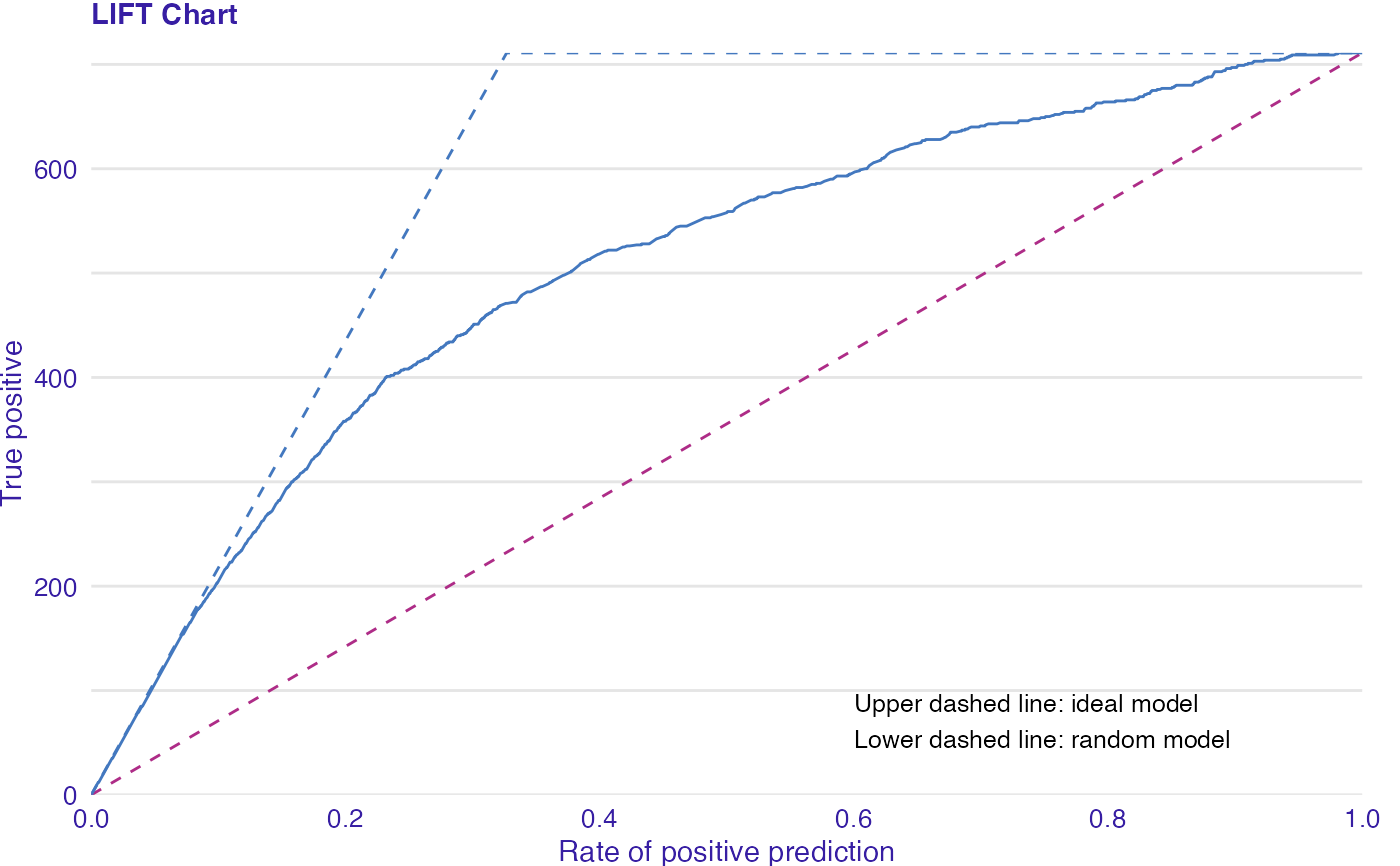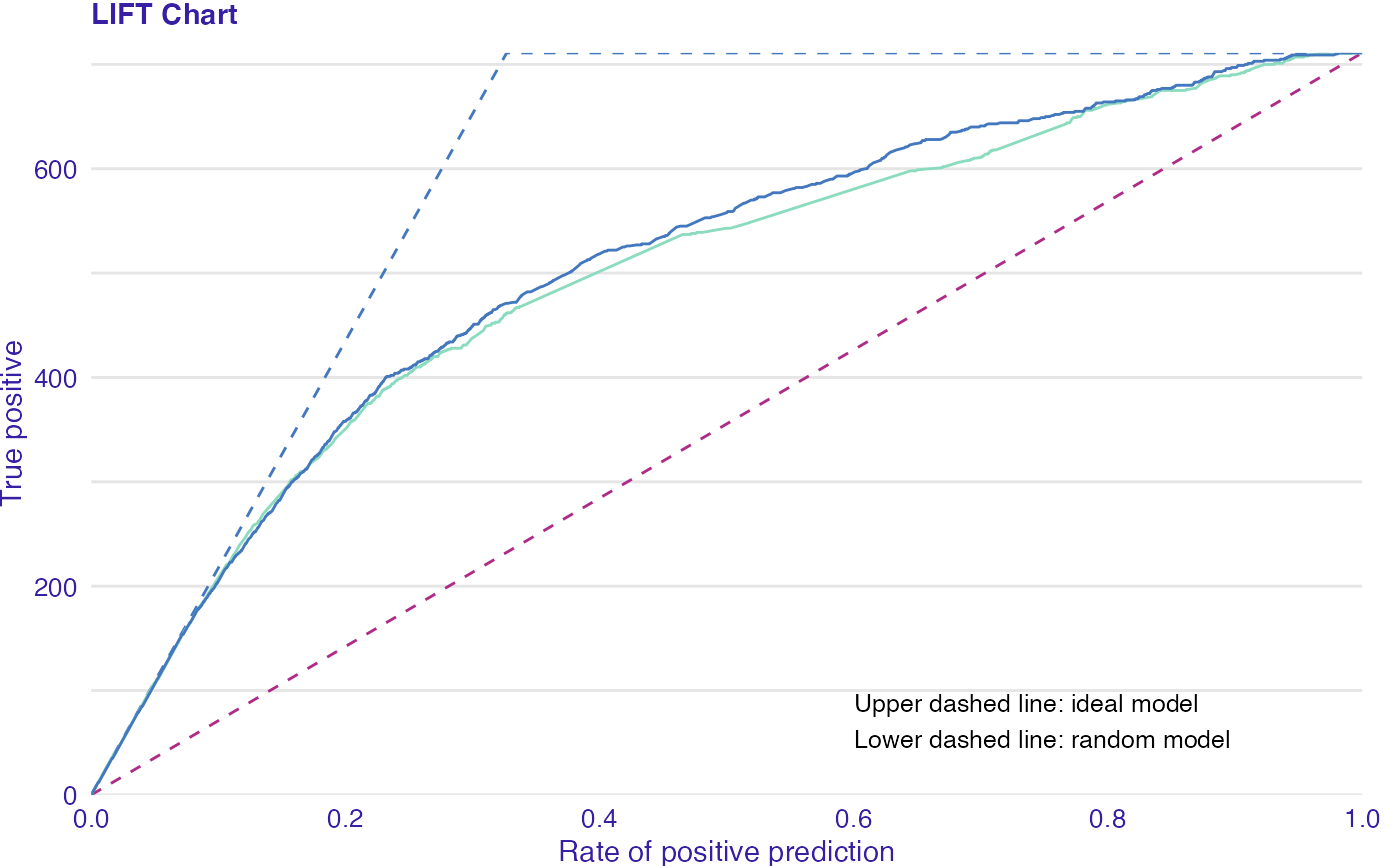LIFT is a plot of the rate of positive prediction against true positive rate for the different thresholds. It is useful for measuring and comparing the accuracy of the classificators.

plot_lift(object, ..., zeros = TRUE)

plotLIFT(object, ...)

## Arguments

object An object of class auditor_model_evaluation created with model_evaluation function. Other auditor_model_evaluation objects to be plotted together. Logical. It makes the lines start from the (0,0) point. By default it's TRUE.

## Value

A ggplot object.

model_evaluation

## Examples

data(titanic_imputed, package = "DALEX")

# fit a model
model_glm <- glm(survived ~ ., family = binomial, data = titanic_imputed)

glm_audit <- audit(model_glm,
data = titanic_imputed,
y = titanic_imputed$survived) #> Preparation of a new explainer is initiated #> -> model label : lm ( default ) #> -> data : 2207 rows 8 cols #> -> target variable : 2207 values #> -> predict function : yhat.glm will be used ( default ) #> -> predicted values : No value for predict function target column. ( default ) #> -> model_info : package stats , ver. 4.1.1 , task classification ( default ) #> -> predicted values : numerical, min = 0.008128381 , mean = 0.3221568 , max = 0.9731431 #> -> residual function : difference between y and yhat ( default ) #> -> residuals : numerical, min = -0.9628583 , mean = -2.569729e-10 , max = 0.9663346 #> A new explainer has been created! # validate a model with auditor eva_glm <- model_evaluation(glm_audit) # plot results plot_lift(eva_glm)plot(eva_glm, type ="lift")model_glm_2 <- glm(survived ~ .-age, family = binomial, data = titanic_imputed) glm_audit_2 <- audit(model_glm_2, data = titanic_imputed, y = titanic_imputed$survived,
label = "glm2")
#> Preparation of a new explainer is initiated
#>   -> model label       :  glm2
#>   -> data              :  2207  rows  8  cols
#>   -> target variable   :  2207  values
#>   -> predict function  :  yhat.glm  will be used (  default  )
#>   -> predicted values  :  No value for predict function target column. (  default  )
#>   -> model_info        :  package stats , ver. 4.1.1 , task classification (  default  )
#>   -> predicted values  :  numerical, min =  0.01618585 , mean =  0.3221568 , max =  0.9628841
#>   -> residual function :  difference between y and yhat (  default  )
#>   -> residuals         :  numerical, min =  -0.9354237 , mean =  -1.981381e-10 , max =  0.9654417
#>   A new explainer has been created!  eva_glm_2 <- model_evaluation(glm_audit_2)

plot_lift(eva_glm, eva_glm_2)plot(eva_glm, eva_glm_2, type = "lift")